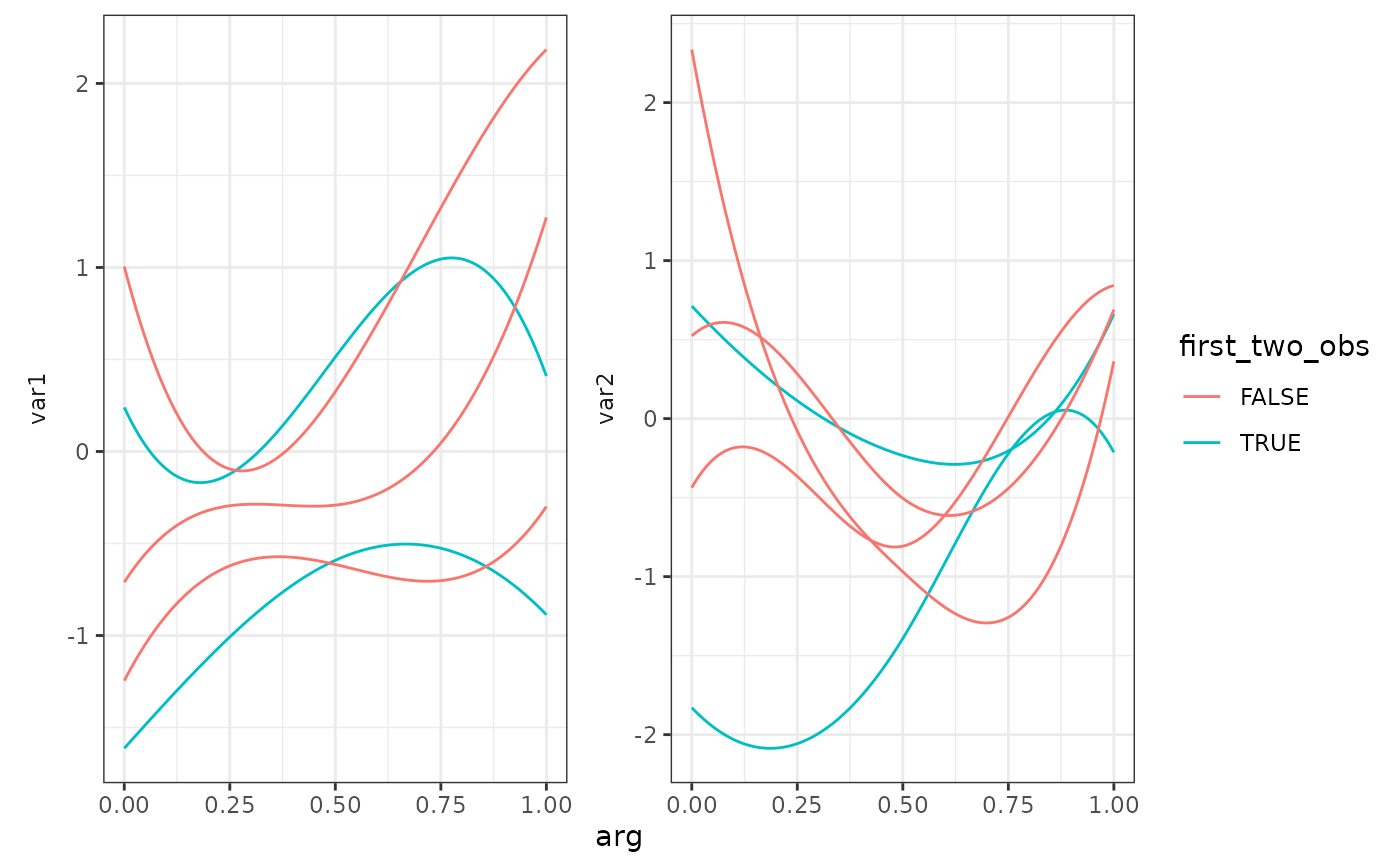Creates a geom layer to plot a Multivariate Functional Data Object with ggplot

geom_mfd(
mapping = NULL,
data = NULL,
mfdobj,
stat = "identity",
position = "identity",
na.rm = TRUE,
orientation = NA,
show.legend = NA,
inherit.aes = TRUE,
type_mfd = "mfd",
...
)

## Arguments

mapping

Set of aesthetic mappings additional to x and y as passed to the function ggplot2::geom:line.

data

A data.frame providing columns to create additional aesthetic mappings. It must contain a column "id" with the replication values as in mfdobj$fdnames[]. If it contains a column "var", this must contain the functional variables as in mfdobj$fdnames[].

mfdobj

A multivariate functional data object of class mfd.

stat

See ggplot2::geom_line.

position

See ggplot2::geom_line.

na.rm

See ggplot2::geom_line.

orientation

See ggplot2::geom_line.

show.legend

See ggplot2::geom_line.

inherit.aes

See ggplot2::geom_line.

type_mfd

A character value equal to "mfd" or "raw". If "mfd", the smoothed functional data are plotted, if "raw", the original discrete data are plotted.

...

See ggplot2::geom_line.

## Value

A geom_line layer to be added to ggplot2::ggplot()in order to plot mfdobj.

## Examples

library(funcharts)
mfdobj <- data_sim_mfd()
ids <- mfdobj\$fdnames[]
df <- data.frame(id = ids, first_two_obs = ids %in% c("rep1", "rep2"))
ggplot() +
geom_mfd(mapping = aes(colour = first_two_obs),
data = df,
mfdobj = mfdobj)